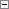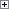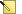Calculates a new date that is based on a date expression and a reference date.

NewDate := CALCDATE(DateExpression [, Date])

Parameters

DateExpression

Type: Text, Code, or DateFormula

The date expression can be any length. The string is interpreted from left to right with one subexpression at a time. The following rules describe the valid syntax of date expressions:

• DateExpression = [<SubExpression>][<SubExpression>][<SubExpression>]
• <SubExpression> = [<Sign>] <Term>
• <Sign> = + | -
• <Term> = <Number><Unit> | <Unit><Number> | <Prefix><Unit>
• <Number> = Positive integer
• <Unit> = D | WD | W | M | Q | Y (D=day, WD=weekday, W=week, M=month, Q=quarter, Y=year)
• <Prefix> = C (C=current)
These production rules show that date expressions consist of zero, one, two, or three subexpressions. Each subexpression consists of an optional sign and a term. The following are some typical examples of terms:

• 30D (30 days; corresponds to <Number><Unit>)
• WD2 (weekday number 2; corresponds to <Unit><Number>)
• CW (current week; corresponds to <Prefix><Unit>)
The internal calendar of Microsoft Dynamics NAV starts on Monday and ends on Sunday. This means that Monday is weekday 1 and Sunday is weekday 7.

A run-time error occurs if the syntax of DateExpression is incorrect.

Date

Type: Date

Use this optional parameter to define a reference date. The default is the current system date.

If you omit this optional value, the current system date is used.Property Value/Return Value

Type: Date

The date that is computed from the reference date and the date expression.Remarks

DateExpression can be a field or variable of type Text or Code, and it can be a field or variable of type DATEFORMULA. The benefit of using a DateFormula field or variable is that the date formula becomes language independent.

The user can enter formulas in the currently selected language. The formula is stored in a generic format in a field or variable. When the formula must be displayed, the actual string that is displayed is converted to the currently selected language.

For example, if a user who has language set to ENG (English) enters the date formula "1W+1D" for one week and one day, then a user who has the language set to FRA (French) sees "1S+1J," and a user who has the language set to ESP (Spanish) sees "1S+1D".

If a date formula is entered with < > delimiters surrounding it, then the date formula is stored in a generic, nonlanguage-dependent format. This makes it possible to develop date formulas that are not dependent on the currently selected language.

For more information about how to calculate the duration between two DateTimes, see Duration Data Type.Example

This code example shows how to use the production rules that were previously described.Copy Code
<CQ+1M-10D>

This should be interpreted as the following: current quarter + 1 month - 10 days.

The DateExpression is composed of the following:

<Prefix><Unit><Sign><Number><Unit><Sign><Number><Unit>Note
The angle brackets (< >) specify that the expression is not translated, regardless of the application language. For more information about multilanguage capabilities with date formulas, see Developing Multilanguage-Enabled Applications.Example

This example shows how to use the CALCDATE function.

This code example requires that you create the following variables and text constants in the C/AL Globals window.

Variable Name DataType Length

Expr1

Text

30

Expr2

Text

30

Expr3

Text

30

RefDate

Date

Not applicable

Date1

Date

Not applicable

Date2

Date

Not applicable

Date3

Date

Not applicable

Text constant ENU value

Text000

'The reference date is: %1 \'

Text001

'The expression: %2 returns %3\'

Text002

'The expression: %4 returns %5\'

Text003

'The expression: %6 returns %7'Copy Code
Expr1 := '<CQ+1M-10D>'; // Current quarter + 1 month - 10 days
Expr2 := '<-WD2>'; // The last weekday no.2, (last Tuesday)
Expr3 := '<CM+30D>'; // Current month + 30 days
RefDate := 052196D;
Date1 := CALCDATE(Expr1, RefDate);
Date2 := CALCDATE(Expr2, RefDate);
Date3 := CALCDATE(Expr3, RefDate);
MESSAGE(Text000 + Text001 + Text002 + Text003,
RefDate, Expr1, Date1, Expr2, Date2, Expr3, Date3);

The message window displays the following text:

The reference date is: 05/21/96

The expression: CQ+1M-10D returns 07/20/96

The expression: -WD2 returns 05/14/96

The expression: CM+30D returns 06/30/96